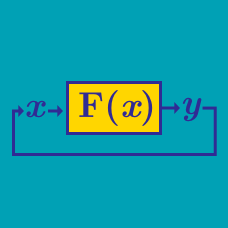Computer Science

# Functions (CS): Level 1 Challenges

Can you identify the function f(x,y) which takes two positive integers as its argument?

 1 2 3 4 5 6 7 8 9 def f(x,y): minimum = min(x,y) maximum = max(x,y) if minimum == 0: return maximum elif minimum == 1: return 1 else: return f(minimum,maximum-minimum) 

What would the following python code print out?

 1 2 3 4 5 6 current_year = 2015 andy_birth = 1984 andy_minus_adaline = 4 adaline = andy - andy_minus_adaline andy = current_year - andy_birth print adalineThe Ackermann function is a computable function which grows very, very quickly as its inputs grow. For example, while $A(1,2),$ $A (2,2),$ and $A(3,2)$ are equal to $4,7,$ and $29,$ respectively, $A(4,2) \approx 2 \times 10^{19728}$.

The Ackermann function can be defined as follows: $A(m,n) = \begin{cases} n+1 & \mbox{if } m = 0 \\ A(m-1, 1) & \mbox{if } m > 0 \mbox{ and } n = 0 \\ A(m-1, A(m, n-1)) & \mbox{if } m > 0 \mbox{ and } n > 0. \end{cases}$

What is the value of $A(3,6)?$

×